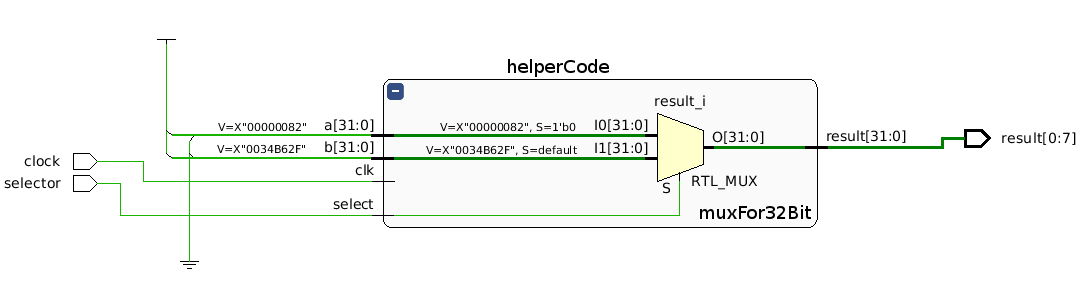In this week, we designed a 32 bit multiplexer in the lab. You can download the manual  for this lab session that sent by our lecturer from this link.

Our purpose is designing a 32 bit multiplexer but our FPGA board has just 8 leds so we could not observe 32 bits. For this problem, we showed just last 8 bits of result via leds on the board. So, we designed a 32 bit multiplexer and another module for get last 8 bits of result from 32 bit multiplexer. Our new module has two inputs (selector, clock) and an output (8 bits of result).

Here are codes:

```
module multiplexer(
input [31:0] a,
input [31:0] b,
input select,
output [31:0] result,
input clk
);
wire [31:0] a,b;
reg [31:0] result;

always @(a,b,select)
begin
if(select==0)
result=a;
else
result=b;
end
endmodule

```

The code above is a design for 32 bit multiplexer, but we can’t observe 32 bit result on FPGA board because of leds count. We need creating a new module for check the code as I said above. In this module, we must get only last eight bits of the result from multiplexer module and observe value of these leds on the FPGA board.

```
module mainCode(
input selector,
input clock,
output [0:7] result
);
wire [0:31] a,b;
assign a='d130;
assign b='d3454511;
wire [0:31] temp;
multiplexer child(a,b,selector,temp,clock);

assign result=8'b11111111 &amp; temp;
endmodule

```

Why we use the clock?

Actually, we don’t need a clock for this design because it is simple. If we have a complex design, we should use a clock for working sync on FPGA board. If we don’t use a clock, some of values from inputs or calculation algorithms may be delayed so the result may be wrong.

Here is, the design:Here is, the code is running on the board: